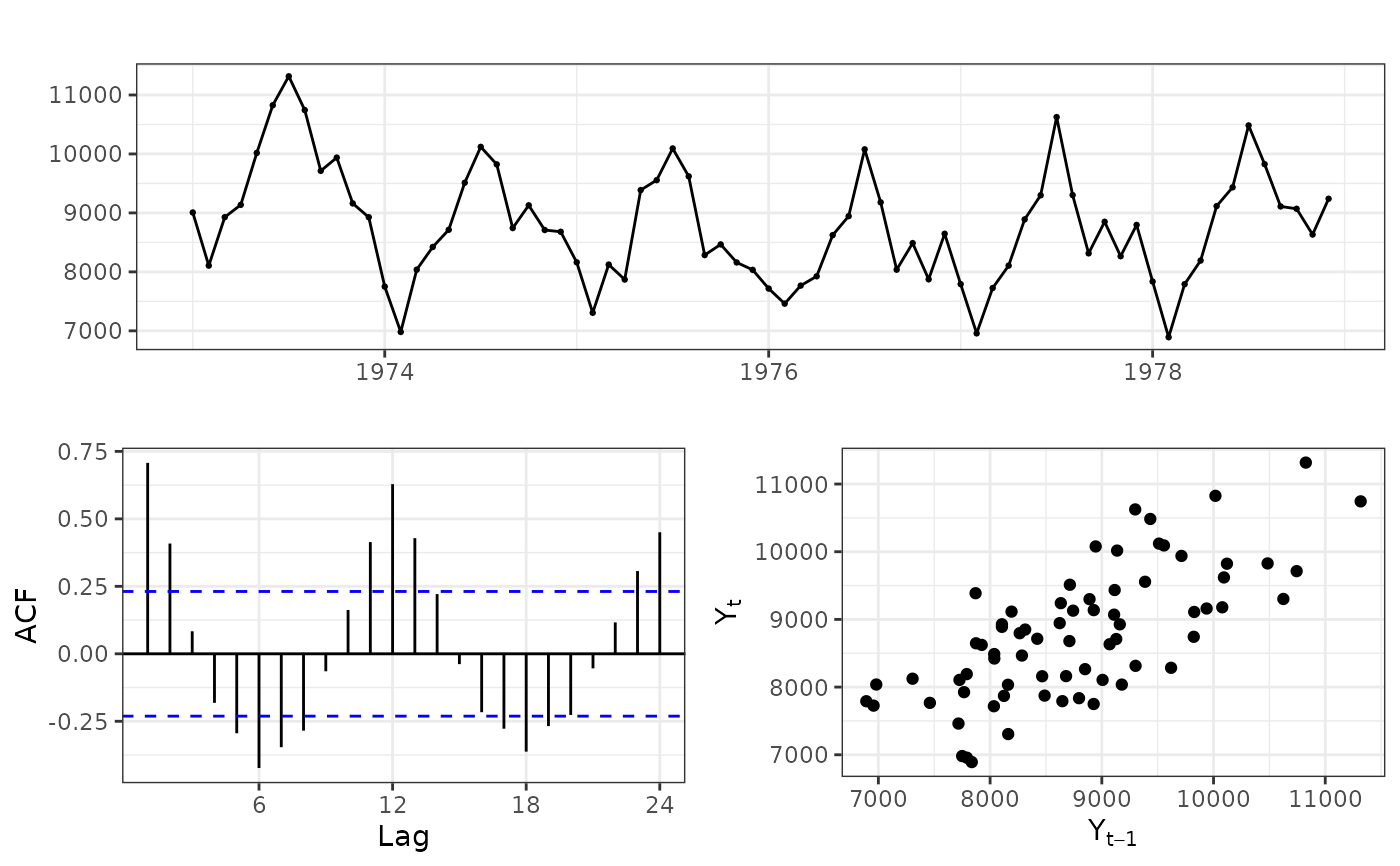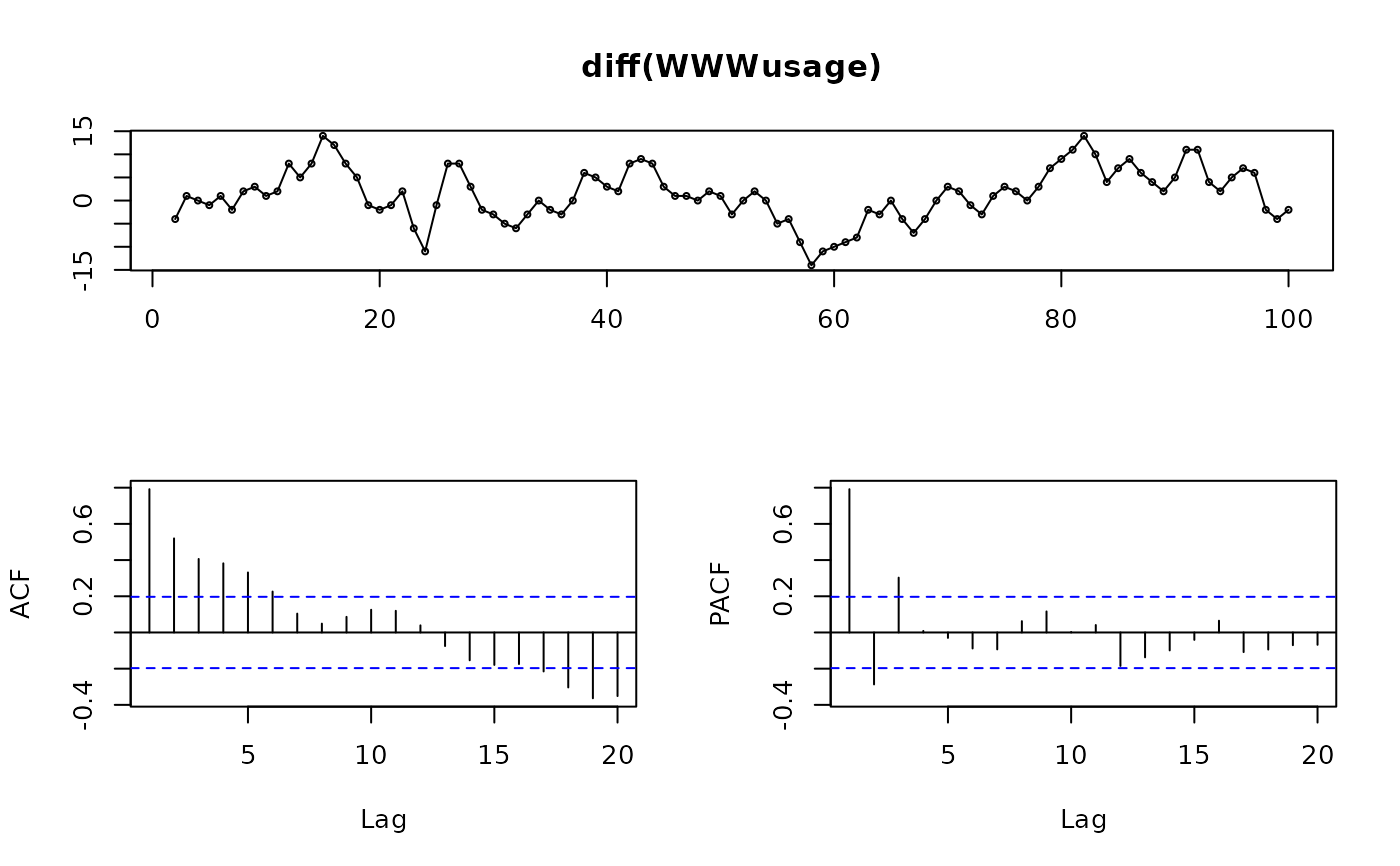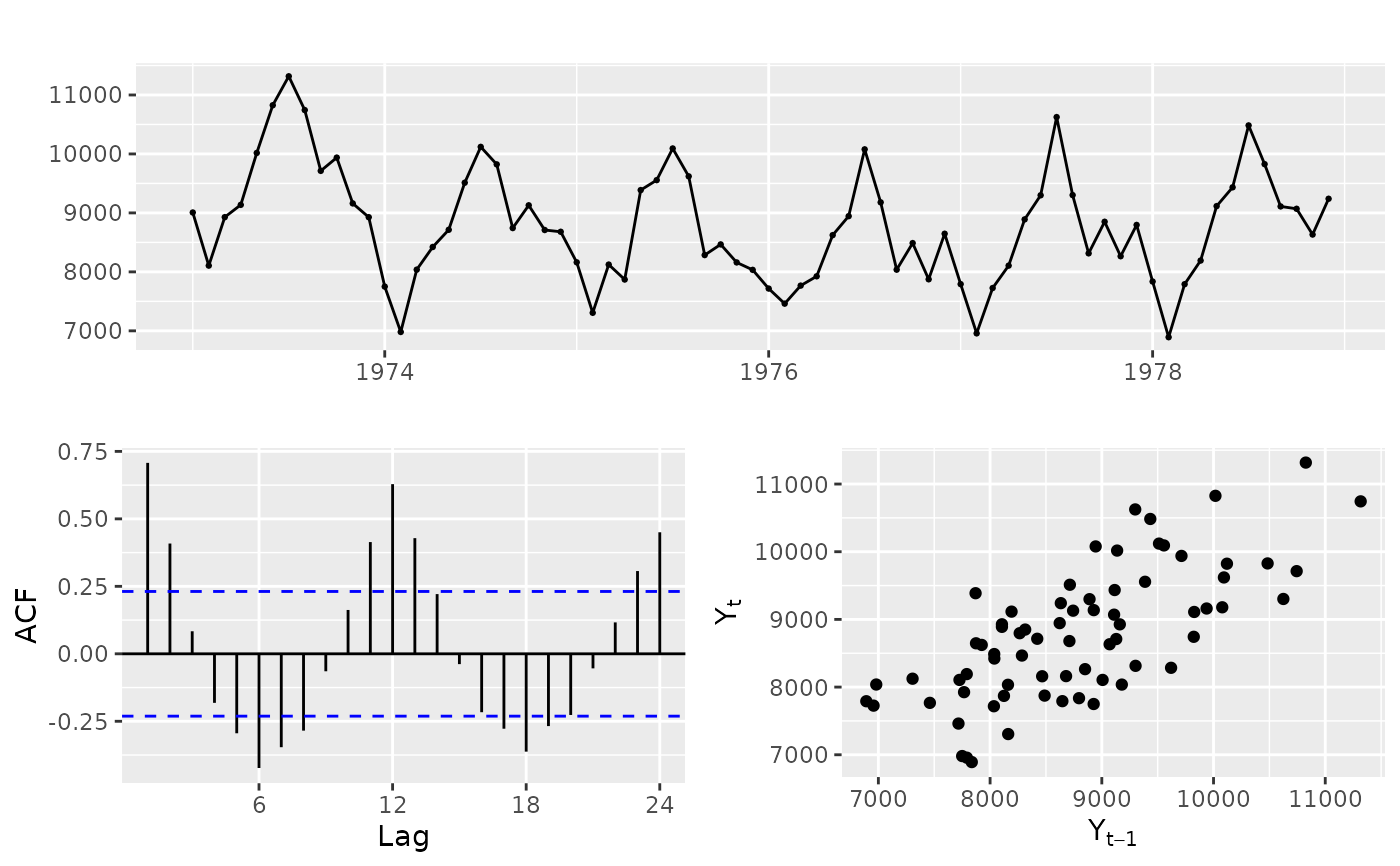Plots a time series along with its acf and either its pacf, lagged scatterplot or spectrum.

ggtsdisplay(
x,
plot.type = c("partial", "histogram", "scatter", "spectrum"),
points = TRUE,
smooth = FALSE,
lag.max,
na.action = na.contiguous,
theme = NULL,
...
)

tsdisplay(
x,
plot.type = c("partial", "histogram", "scatter", "spectrum"),
points = TRUE,
ci.type = c("white", "ma"),
lag.max,
na.action = na.contiguous,
main = NULL,
xlab = "",
ylab = "",
pch = 1,
cex = 0.5,
...
)

## Arguments

x a numeric vector or time series of class ts. type of plot to include in lower right corner. logical flag indicating whether to show the individual points or not in the time plot. logical flag indicating whether to show a smooth loess curve superimposed on the time plot. the maximum lag to plot for the acf and pacf. A suitable value is selected by default if the argument is missing. function to handle missing values in acf, pacf and spectrum calculations. The default is na.contiguous. Useful alternatives are na.pass and na.interp. Adds a ggplot element to each plot, typically a theme. additional arguments to acf. type of confidence limits for ACF that is passed to acf. Should the confidence limits assume a white noise input or for lag $$k$$ an MA($$k-1$$) input? Main title. X-axis label. Y-axis label. Plotting character. Character size.

None.

## Details

ggtsdisplay will produce the equivalent plot using ggplot graphics.

Hyndman and Athanasopoulos (2018) Forecasting: principles and practice, 2nd edition, OTexts: Melbourne, Australia. https://otexts.com/fpp2/

plot.ts, Acf, spec.ar

Rob J Hyndman

## Examples

library(ggplot2)
ggtsdisplay(USAccDeaths, plot.type="scatter", theme=theme_bw())tsdisplay(diff(WWWusage))ggtsdisplay(USAccDeaths, plot.type="scatter")